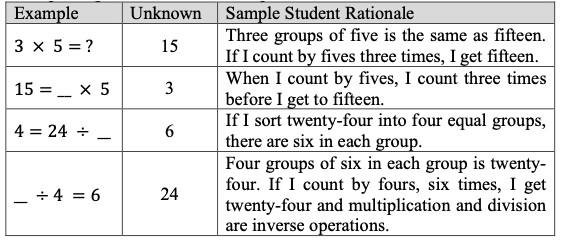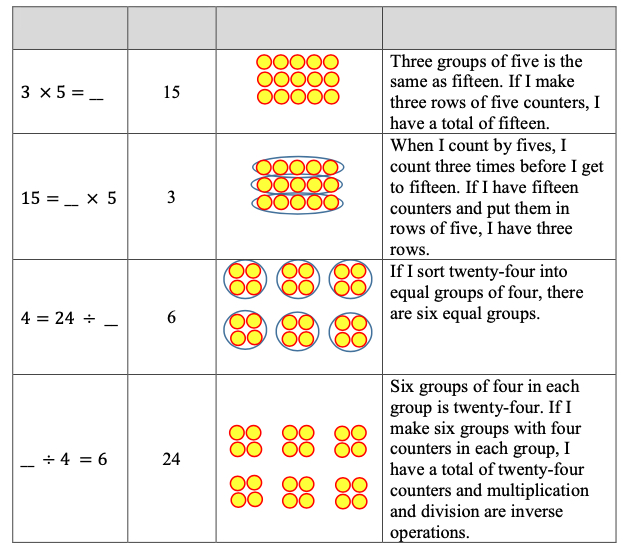# Standard #: MA.3.AR.2.3

This document was generated on CPALMS - www.cpalms.org

Determine the unknown whole number in a multiplication or division equation, relating three whole numbers, with the unknown in any position.

### Clarifications

Clarification 1: Instruction extends the development of algebraic thinking skills where the symbolic representation of the unknown uses any symbol or a letter.
Clarification 2: Problems include the unknown on either side of the equal sign.
Clarification 3: Multiplication is limited to factors within 12 and related division facts. Refer to Situations Involving Operations with Numbers (Appendix A).

### General Information

Subject Area: Mathematics (B.E.S.T.)
Strand: Algebraic Reasoning
Status: State Board Approved

• Equation
• Expression

### Vertical Alignment

Previous Benchmarks

Next Benchmarks

### Purpose and Instructional Strategies

The purpose of this benchmark is for students to find an unknown value represented by a symbol or letter in a multiplication or division equation, continuing the work from Grade 2, where students found unknown values in addition and subtraction equations.
• Instruction that emphasizes the relationship between related facts in a fact family helps students use known values to solve for unknown values. For example, a fact family could be used to help students determine the unknown value in the equation 72 ÷ ? = 9 (MTR.5.1).
72 ÷ ? = 9
72 ÷ 9 =?
9 × ? = 72
? × 9 = 72
• Students can use any of these related facts to determine that the unknown value is 8. Teachers should encourage students to use such equations to justify their solutions (MTR.6.1).
• In the primary grades, students used fact families to find missing addends and understand the relationship between addition and subtraction.
• Understanding and using related facts to solve for unknown values is an important algebraic understanding for using inverse operations to solve equations in future mathematics courses (MTR.5.1).

### Common Misconceptions or Errors

• By Grade 3, many students expect the solutions of equations to be an expression on the right side of the equal sign. When students determine unknown values in multiplication or division equations, give examples with the product or quotient on the left side.

### Strategies to Support Tiered Instruction

• Instruction includes opportunities to explore the meaning of the equal sign within the context of multiplication and division. The teacher provides clarification that the equal sign means “the same as” rather than “the answer is,” supporting the understanding that the product and quotient can be on either the left or the right side of the equal sign. Multiple examples are provided for students to solve for the unknown with the product or quotient on both the left and right sides of the equation. The teacher uses the same equations written in different ways to reinforce the concept.
• For example, the teacher shows the following equations, asking students to solve for the unknown. Students explain why each equation is true after solving, repeating with additional examples.• Teacher provides opportunities to explore the meaning of the equal sign within the context of multiplication and division using visual representations (e.g., counters, drawings, base-ten blocks) to represent the equations. The teacher provides clarification that the equal sign means “the same as” rather than “the answer is,” supporting the understanding that the product and quotient can be on either the left or the right side of the equal sign. Multiple examples are provided for students to solve for the unknown with the product or quotient on both the left and right sides of the equation, using the same equations written in different ways to reinforce the concept.
• For example, the teacher shows the following equations, asking students to solve for the unknown and explain why each equation is true after solving. Students use counters, drawings, or base-ten blocks to represent the equation, repeating with additional operations.Sam is having trouble deciding whether the value of $n$ that makes the equation below true is 4 or 36. Which number is correct? Show your thinking using an equation or array.
3 = $n$ ÷ 12

### Instructional Items

Instructional Item 1

What value of $n$ makes the equation below true?
$n$ ÷ 6 = 5

Instructional Item 2

What is the value of the unknown number in the equation 7 × $n$ = 56?

*The strategies, tasks and items included in the B1G-M are examples and should not be considered comprehensive.

#### Related Courses

 Course Number1111 Course Title222 5012050: Grade Three Mathematics (Specifically in versions: 2014 - 2015, 2015 - 2022, 2022 and beyond (current)) 7712040: Access Mathematics Grade 3 (Specifically in versions: 2014 - 2015, 2015 - 2018, 2018 - 2022, 2022 and beyond (current)) 5012055: Grade 3 Accelerated Mathematics (Specifically in versions: 2019 - 2022, 2022 and beyond (current)) 5012015: Foundational Skills in Mathematics 3-5 (Specifically in versions: 2019 - 2022, 2022 and beyond (current))

#### Related Access Points

 Access Point Number Access Point Title MA.3.AR.2.AP.3 Determine the unknown whole number in a multiplication or division equation, relating three whole numbers, with the product or quotient unknown (e.g., 2 × 5 = __, 10 ÷ 5 = __). Multiplication may not exceed two single-digit whole numbers and their related division facts.

#### Formative Assessments

 Name Description Multiplication and Division Equations Students are given multiplication and division equations within 100 and asked to find the missing numbers. The missing numbers are presented in all positions. Missing Numbers In Multiplication Equations Students are given multiplication equations with products within 50 and are asked to find missing numbers. The missing numbers are presented in all positions. Missing Numbers In Division Equations Students are given division equations involving numbers within 50 and are asked to find missing numbers. The missing numbers are presented in all positions. Find the Unknown Number Students are given multiplication and division equations within 50 and are asked to find missing numbers. The missing numbers are presented in all positions.

#### Lesson Plans

 Name Description Cupid's Carnival Rides In this lesson, students will look at different carnival rides and will determine which ride will make the most profit by looking at factors such as number of tickets per ride, the cost per ticket, the length of the ride, the number of hours the ride is open and the cost to operate the ride. Students will need to use different operations in order to solve the tasks and will be required to do multi-steps.Model Eliciting Activities, MEAs, are open-ended, interdisciplinary problem-solving activities that are meant to reveal students’ thinking about the concepts embedded in realistic situations. Click here to learn more about MEAs and how they can transform your classroom. Arrays Show the Way to the Multiplication Chart This is an introductory lesson to explore the use of arrays to solve multiplication problems. Students build arrays and save the arrays in a class Multiplication Chart. They learn to use arrays to find products and factors, and by placing them in the Multiplication Chart, they learn how to read the chart. They learn how to write equations to represent situations that are modeled with arrays. An overall theme is the organization of the multiplication chart and how it includes arrays within. Giddy Up, Round Up: Relating Division to Multiplication In this lesson, students will learn to solve division problems by relating them to multiplication facts. Practice materials focus on the 6's and 8's multiplication facts. Tasty Algebra: Using toasted O cereal to find the missing factor in a multiplication equation. In this lesson students will use Cheerios to solve multiplication equations relating 3 whole numbers from word problems that include missing factors ranging from one through ten. Students will also argue the validity of multiplication equations that include missing factors and products with corresponding word problems.

#### Teaching Idea

 Name Description A Square of Numbers (problem to solve using addition, subtraction, multiplication, division) A problem for students to solve using students' understanding of the operations of addition, subtraction, multiplication, and division. The problem presents an opportunity for students to appreciate the value of approaching a solution in a systematic way using what they know about the structure of operations.
Printed On:9/24/2023 3:28:09 AM
Print Page | Close this window